# UNIT 47: Gating Design Wizard

The SOLIDCast Gating Design Wizard is an integrated function of the SOLIDCast system. The Gating Design Wizard allows you to size sprues, runners and gates for either horizontally-parted or vertically-parted molds, using generally recognized methods for calculation of these gating system components.

# Basic Theory of Gating Design

The basic idea in gating design is actually very simple. One starts by estimating the Fill Time required for a casting. This may be based on experience with certain types of castings. It may also be based on a calculation involving the weight poured, the type of alloy and the critical section thickness to be poured; the Gating Design Wizard contains such a calculation as a built-in function.

Knowing the Fill Time, and given the weight and density of the casting, it is possible to calculate a volumetric flow rate (cubic inches/sec or cc/sec) using the formula:

Volume
Flow rate =
──────

Fill Time

Again, the Gating Design Wizard contains this formula as a built-in calculation function.

Next, it is necessary consider how far the metal will fall when it is poured, which gives a metal velocity (using Newton's laws of motion for a free-falling body). Knowing the velocity and the volumetric flow rate, the cross-sectional area of flow required can be calculated (see the equations on the following pages). It is then necessary to adjust this flow area for friction loss or shape factors, and finally to apportion this area so that there is the desired rate of flow at all of the various gates into the casting. It is also necessary to establish the “choke” point of the gating system, so that elements downstream (or upstream) from the choke can be oversized sufficiently to avoid excessive velocities and maintain the choke (the point of maximum velocity) at the correct point in the gating system. This will generally ensure the correct rate of flow in all portions of the gating system, with liquid metal delivered at the required flow rate into the casting cavity.

It is suggested that the user consult further references for complete design of gating components and systems, such as the AFS Handbook on Basic Principles of Gating, and papers by Roger Brown of Disamatic.

The Gating Design Wizard: Horizontal Gating Design

For design of Horizontal Gating Systems, the Gating Design Wizard will calculate sizes of sprues, runners and gates to deliver the liquid metal into the casting cavity. These components are shown in the following example: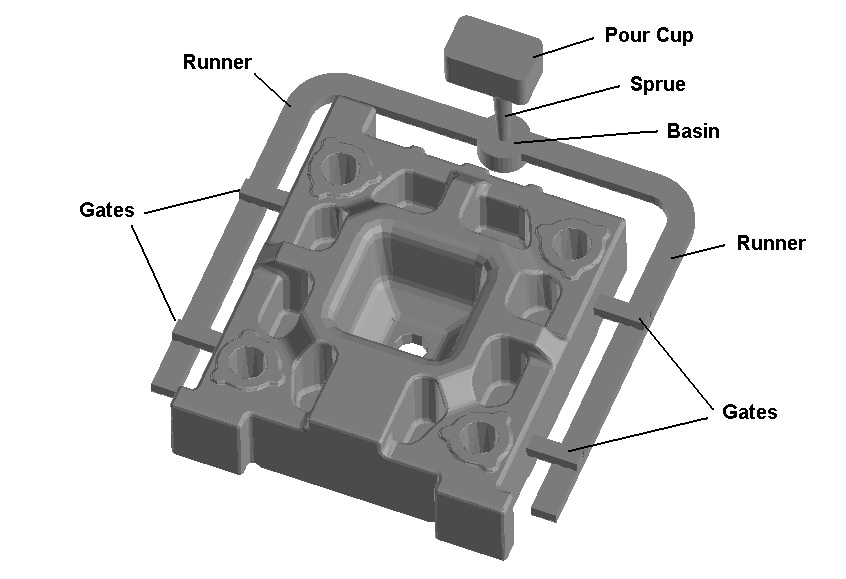Typical Gating System Components

In order to use Gating Design, you must have meshed and simulated a model of the casting. This model may include risers and gating, or it may include just the casting. In the case of a horizontal gating system, if you have modeled only the casting, you will probably want to adjust the weight upward to reflect the estimated total pour weight (including risers and gating).

To start the Gating Design Wizard, first double-click on the Simulation icon on the SOLIDCast Project Tree (Step 1 in the picture below). This will cause the Simulation Status window to appear. Next, close this window (Step 2).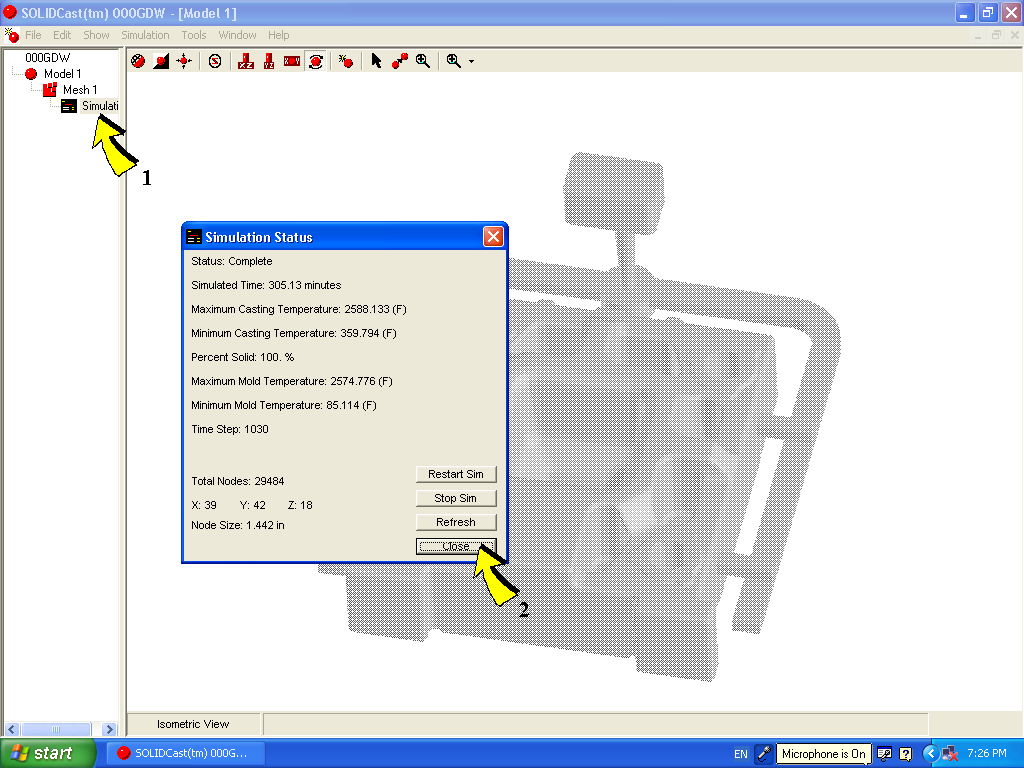Now, select Simulation from the SOLIDCast main menu (Step 3) and then select Gating Design Wizard (Step 4).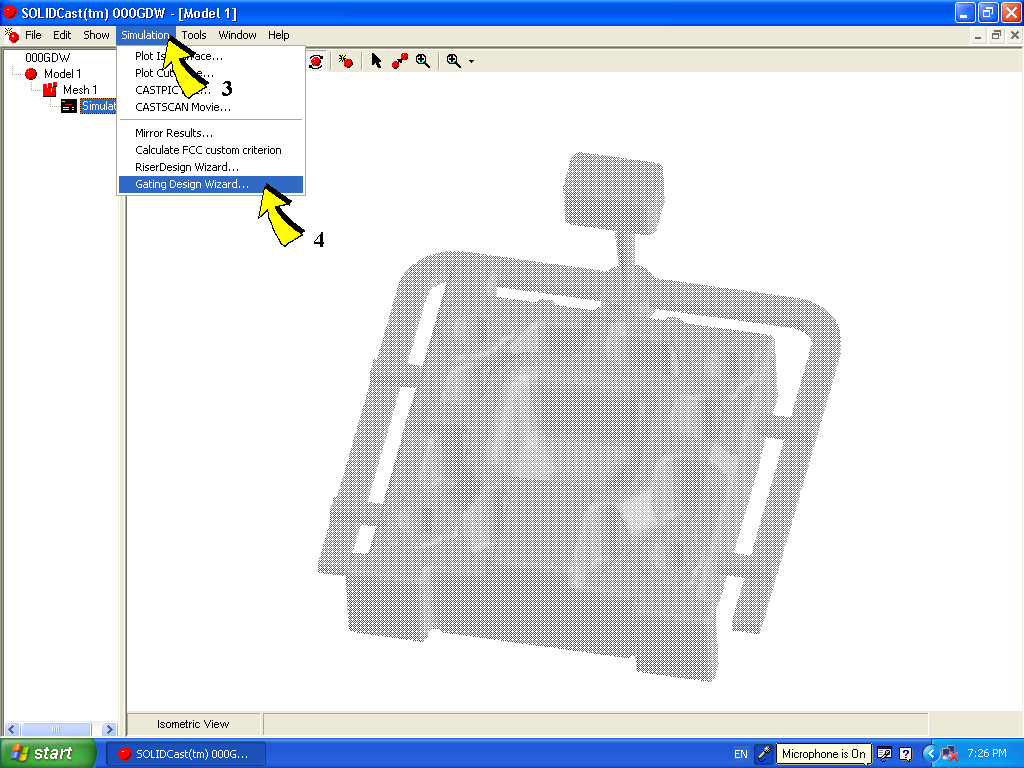This will bring up the initial Gating Design Wizard window, which appears as follows: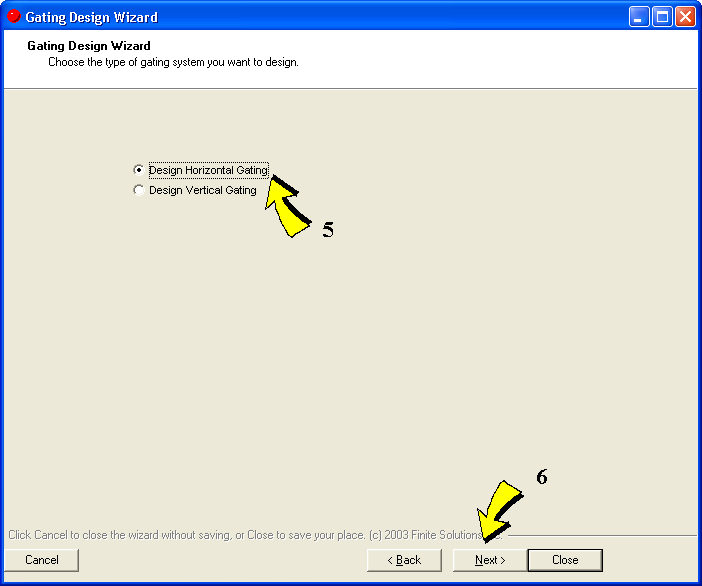Here we can select whether to design a Horizontal Gating System or a Vertical Gating System. In this case, we will select Design Horizontal Gating (Step 5) and then select Next (Step 6).

This will cause the initial Horizontal Gating design screen to appear, as follows: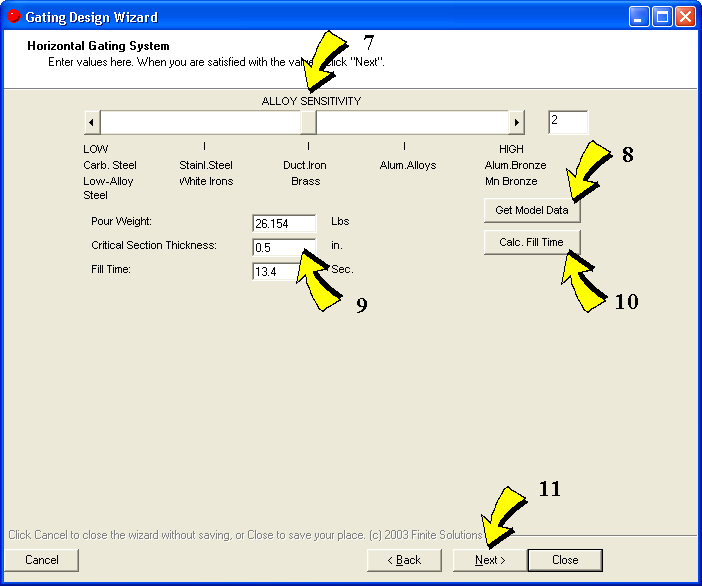Gating Design allows the user to enter a required fill time for a casting, or the program will calculate an "Optimal Fill Time"(OFT) based on weight, critical section thickness and the alloy sensitivity.

In order to have the wizard calculate an OFT, then the following must be specified:

Alloy Sensitivity - This is specified with the slider bar at the top of the screen (Step 7). This refers to the sensitivity of various alloys to form oxides during the pouring process. Alloys which are less sensitive (low value of this factor) may be poured more quickly. Alloys which are more sensitive to oxide formation should be poured more slowly to avoid excessive turbulence which may expose more of the metal surface to oxygen, thus forming and entraining the oxides as inclusions in the finished casting.

Pour Weight - For horizontal-parted castings, this refers to the poured weight (total weight of casting plus rigging). For vertical castings, this weight is intended to be the weight of one casting without rigging. If you press the Get Model Data button (Step 8), the program will extract the weight from the simulation files. Keep in mind that, if the model does not include gating or risering, you may need to increase the displayed weight. The exact value of the weight is not highly critical, since the OFT formula uses the cube root of the weight to estimate fill time.

Critical Section Thickness - This refers to the thickness of the thinnest section of the casting, i.e., that section which is most likely to misrun. This is what Gating Design uses for its Optimal Fill Time calculation. Enter this value as shown in Step 9.

Once the above parameters have been entered, the program will calculate and display the OFT. This is performed by pressing the
Calc. Fill Time button (Step 10).

As mentioned, the user may also enter his/her own Fill Time as an alternative to the OFT calculation. This may sometimes be the case when process constraints (such as an automatic pouring cycle) dictate a specific pour time or pour rate. To enter your own Fill Time, just enter a value (seconds) into the field labeled
Fill Time.

Now, press the Next button to advance to the next design screen (Step 11).

Once a fill time and a weight have been established, then the program has the initial information required to begin figuring flow requirements. First, a mass flow rate is calculated (weight divided by fill time). Then, using the density of the metal from the
SOLIDCast model, this is converted to a volume flow ate (cubic inches or cc per second).

The next piece of information required for gating calculations is the height through which the metal will drop. In the case of horizontal gating, this is the effective height of the sprue (the vertical pipe down which the metal initially flows). For vertical gating, this may be the cumulative height from the top of the mold down to each component. In any case, the velocity of the metal after falling through this height can be calculated from a fairly simple relationship:

V = √(2gH)

Where
V = velocity
g = acceleration of gravity
H = height through which the liquid has fallen

This formula is based on basic Newtonian physics, and describes the velocity of any body freefalling in a gravitational field.

Now, given the known velocity and the known volumetric flow rate, the cross-sectional area of flow of the liquid metal can be calculated simply from the following equation:

Volumetric Flow Rate
Flow Area =
────────────--
Velocity

This is the basic calculation which is used in gating design.

When calculating flow areas, consideration must also given to shape efficiencies and friction losses. According to research, for example, a square tapered sprue has an efficiency of around 74%; this means that an area calculated per the above formula must be increased by a factor of (1/0.74) or 1.351 to account for the energy losses associated with flowing through this type of shape. Also, in flowing through runner systems, the liquid metal loses energy through friction with the channel walls. This friction loss, which is usually expressed as a percentage, must be compensated for by increasing the area of the downstream runner segments.

Another concept which is used in horizontal gating design is that of the "gating ratio". This is the ratio of the area of flow at three different points of the gating system: the sprue (the vertical pipe where the metal initially runs down); the runner (the horizontal passages through which the metal runs to be delivered to the gates); and the gates (the passages through which the metal actually enters the mold cavity). This is usually expressed in the form of whole numbers, giving the ratio of the area of each of these points as S:R:G. For example, if the gating ratio is given as 1:4:4 then the total cross-sectional area of the runners will be 4 times that of the area at the base of the sprue, and the total cross-sectional area of the gates will be equal to that of the runners (4 times the area at the base of the sprue).

The "choke" is defined as the location within the gating system of the minimum cross-sectional area. In a system with a ratio of 1:4:4, the choke area is at the bottom of the sprue. In a system with a ratio of 4:8:3, then the choke is at the gates (the last number is the minimum). A gating system in which the choke is located at the gates is known as a "pressurized system"; this refers to the fact that there will be pressure behind the gates due to their small area, and the velocity through the gates into the casting will be relatively high. If the choke is at the base of the sprue, then this is a "non-pressurized" system and velocity at the gates will be relatively lower (due to the larger cross-sectional area at the gates). In general, non-pressurized systems are recommended for horizontal gating design.

The following window should be displayed on your screen at this point: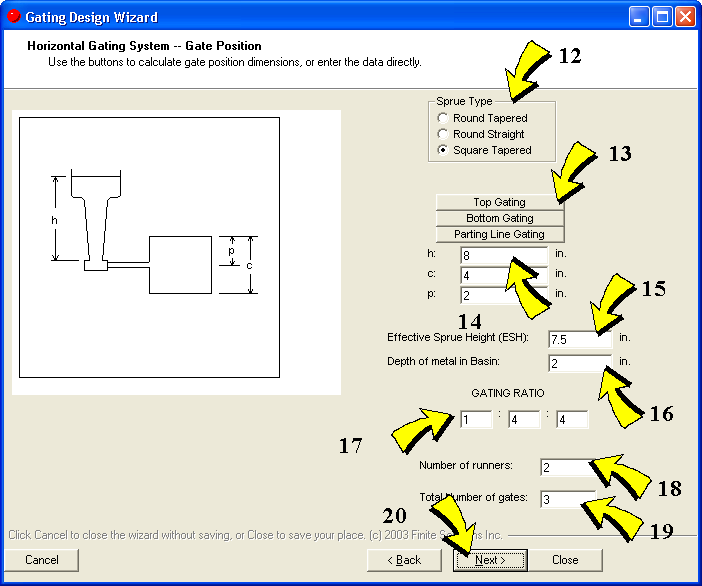The Sprue Type should be selected from the options shown (Step 12). This establishes the efficiency factor to be applied to the area calculation for the sprue.

For the Horizontal Gating Design program, after calculating or entering the Fill Time, the user needs to an Effective Sprue Height (ESH). The ESH may be calculated based on dimensions of the sprue/gate/casting height by selecting the type of gating system (Top, Bottom or Parting Line) as shown at Step 13, then entering appropriate dimensions (Step 14). This will calculate and display the
ESH (Step 15). Note that, if the metal is poured directly into the sprue and not into a pouring basin, then the additional height of the ladle above the top of the mold should be added to the ESH, since this height is used to establish the velocity of the metal after falling to the bottom of the sprue.

The user then must enter the
Depth of metal in Basin at the bottom of the sprue (Step 16). A basin at this location is used to absorb the initial surge of liquid with a minimization of splashing.

The
Gating Ratio is entered next (Step 17), a set of three numbers as described above.

The Number of runners leading away from the base of the sprue (Step 18), and the Total number of gates fed from this sprue (Step 19) must also be entered. At this point, the click the Next button (Step 20) to tell the system to perform the gating calculations and design the individual gating components.

The following window will now appear: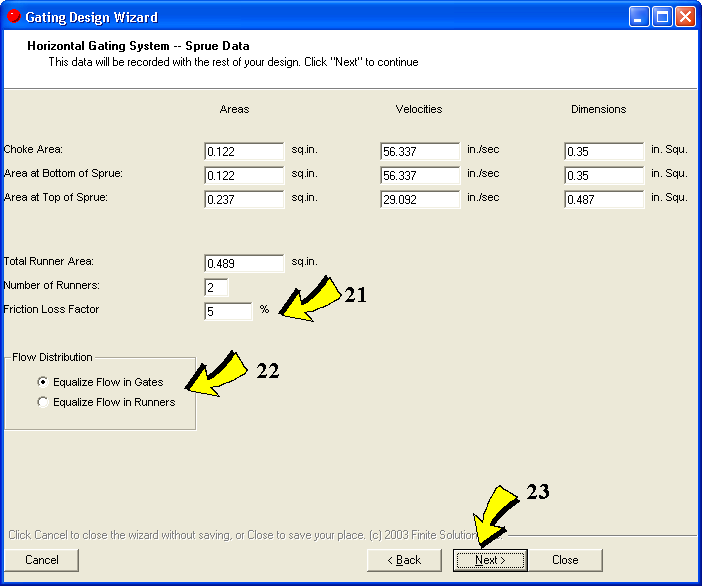This screen shows the choke area, the area at the bottom of the sprue and the area at the top of the sprue, based on the data and calculations as described above. Also shown on this screen are the total required runner area, number of runners and the Friction Loss Factor. The Friction Loss Factor will default to 5% when the program is first run, but it may be modified by the user as Step 21 (for example, some people use 10% as a Friction Loss Factor rather than 5%).

On this screen, you can also select whether to equalize flow through the gates (all gates have equal flow), or whether to equalize flow in the runners (all runners have equal flow, regardless of the number of gates they feed). This is selected as Step 22. Probably the more common practice is to equalize flow through the gates.

Note that for runners which feed multiple gates, in order to equalize flow in the gates it is common practice to "step down" the runner, i.e., reduce its cross-sectional area after each gate in order to equalize the flow to each gate. The amount by which each section is reduced is equal to the area of the preceding gate.

Now press the Next button (Step 23).

The Runner and Gate Design window will now appear as follows: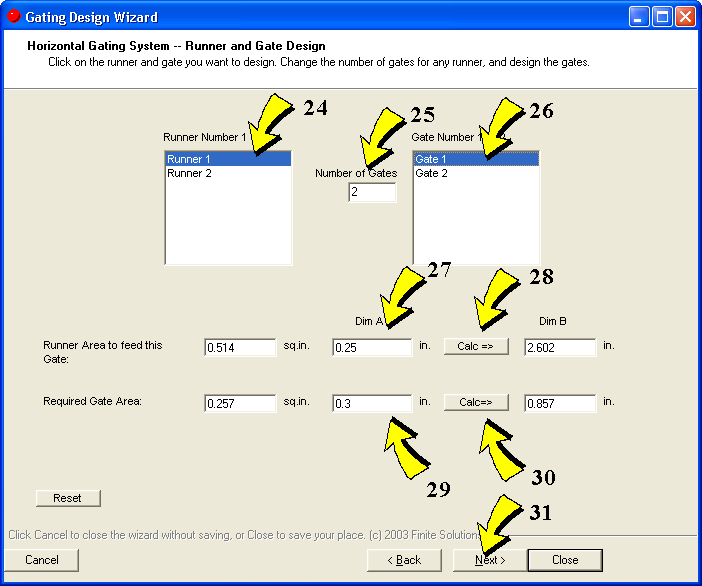This screen will calculate and display the required size for each gate and each section of runner.

First of all, in the left-hand window will be displayed a list of runners (depending on how many runners were previously specified). Select a runner from this list by clicking and highlighting on a runner (Step 24).

Now, enter the number of gates to be fed from this runner (Step 25).

A list of the gates along this runner will be displayed. Select these gates, one at a time (Step 26).

Each time a gate is selected, the dimensions for that gate and the required runner size to feed the gate will be displayed below. Assuming that the runner is rectangular, you can enter Dimension A (Step 27), click the Calc button (Step 28) and the other dimension of the runner will be calculated and displayed.

The same information is displayed for the gate. Enter Dimension A for the gate (Step 29), click the Calc button (Step 30) and the other dimension of the runner will be calculated and displayed.

Note that the runner cross-section is reduced for each subsequent gate along a runner, and also that the area of both the runner and the gate have been increased by the friction loss factor to compensate for the energy loss associated with friction. You can keep selecting subsequent gates along the runner (Step 26) until all of the gates and all sections of this runner have been designed. Once this runner is finished, you can select the next runner to design (Step 24) and perform the same operations to design all gates and runner sections for this runner. This process continues until all runners and all gates have been defined.

As an optional final step, you can review all data by pressing the Next button (Step 31) which will bring up the following screen: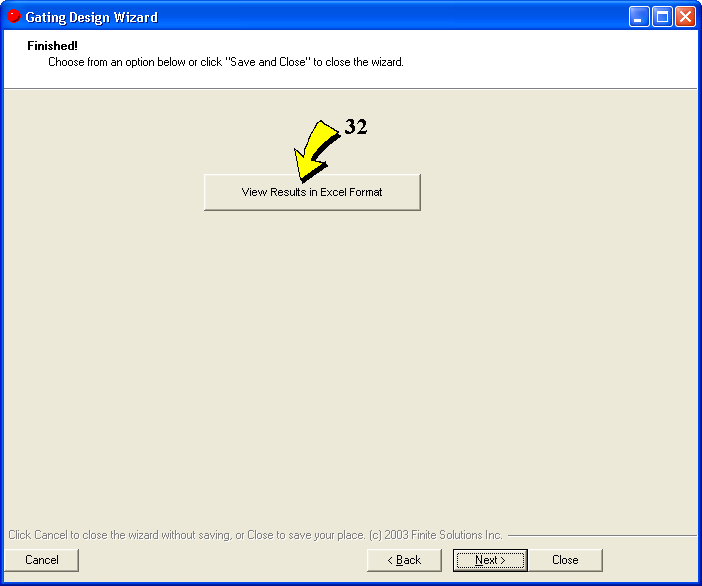By clicking on the button labeled View Results in Excel Format, you can see this data in a spreadsheet format. This assumes that you have Microsoft Excel installed on your computer.
The Gating Design Wizard: Vertical Gating Design

In order to use Vertical Gating Design portion of the Wizard, you must have meshed and simulated a model of the casting. For vertical gating systems, the model should include only one casting plus its risers, without gating components.

To start the Gating Design Wizard, first double-click on the Simulation icon on the SOLIDCast Project Tree (Step 1 in the picture below). This will cause the Simulation Status window to appear. Next, close this window (Step 2).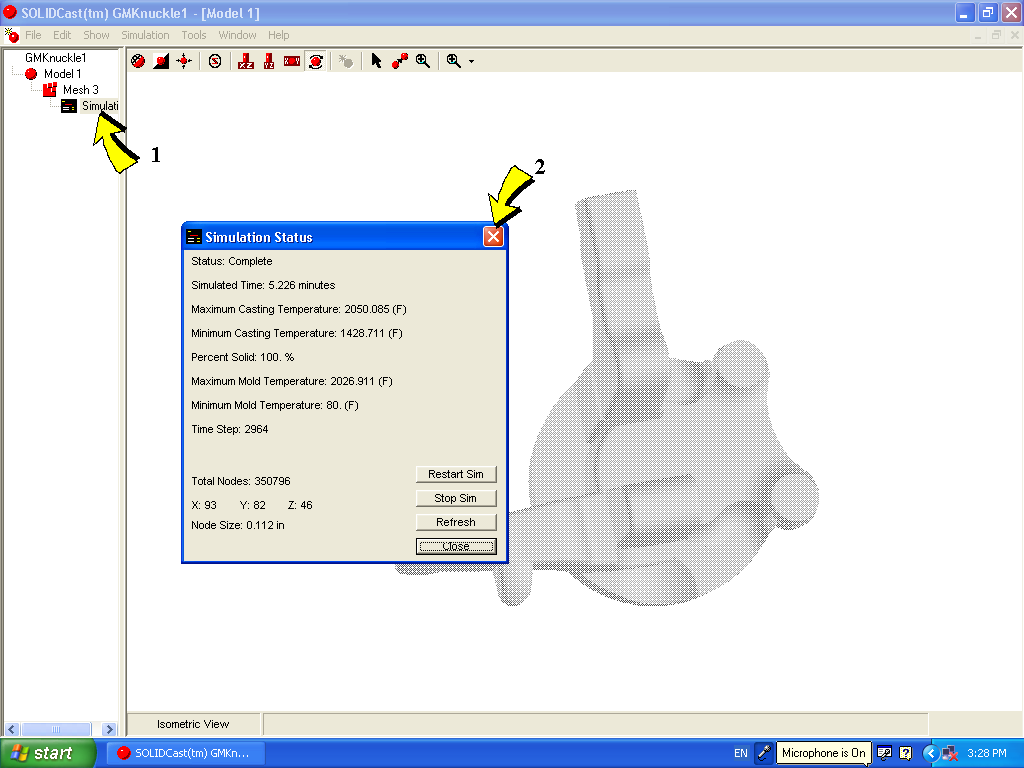Now, select Simulation from the SOLIDCast main menu (Step 3) and then select Gating Design Wizard (Step 4).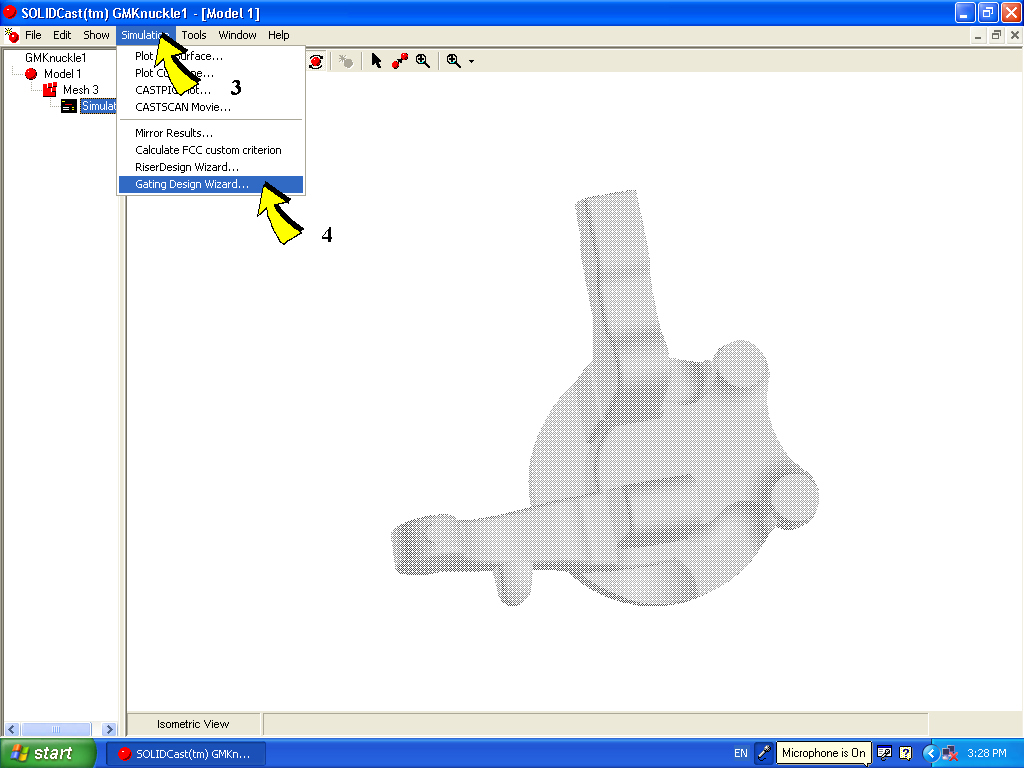This will bring up the initial Gating Design Wizard window, which appears as follows: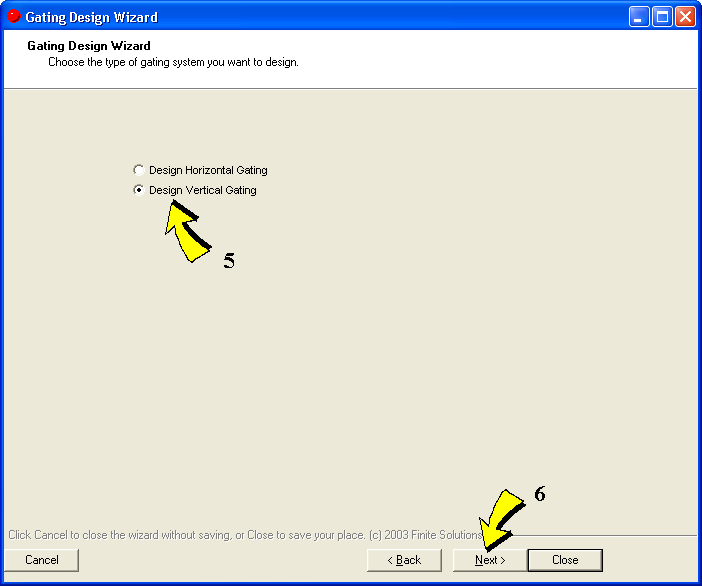Here we can select whether to design a Horizontal Gating System or a Vertical Gating System. In this case, we will select Design Vertical Gating (Step 5) and then select Next (Step 6).

This will cause the initial Vertical Gating design screen to appear, as follows: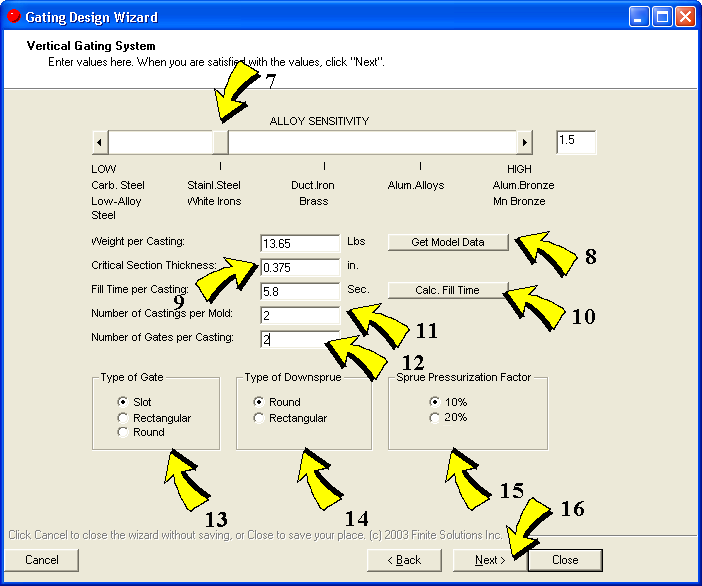Gating Design lets you enter a required fill time for a casting, or the program will calculate an "Optimal Fill Time"(OFT) based on weight, critical section thickness and the alloy sensitivity.

To have the wizard calculate an OFT, then the following must be specified:

Alloy Sensitivity - This is specified with the slider bar at the top of the screen (Step 7). This refers to the sensitivity of various alloys to form oxides during the pouring process. Alloys which are less sensitive (low value of this factor) may be poured more quickly. Alloys which are more sensitive to oxide formation should be poured more slowly to avoid excessive turbulence which may expose more of the metal surface to oxygen, thus forming and entraining the oxides as inclusions in the finished casting.

Weight per Casting - For vertical castings, this weight is intended to be the weight of one casting without gating. If you press the "Get Model Data" button (Step 8), the program will extract the weight from the simulation files. Keep in mind that, if desired, you can adjust the displayed weight. The exact value of the weight is not highly critical, since the OFT formula uses the cube root of the weight to estimate fill time.

Critical Section Thickness - This refers to the thickness of the thinnest section of the casting, i.e., that section which is most likely to misrun. This is what Gating Design uses for its Optimal Fill Time calculation. Enter this value as shown in Step 9.

Once the above parameters have been entered, the program will calculate and display the OFT. This is performed by pressing the
Calc. Fill Time button (Step 10).

As mentioned, you may also enter your own Fill Time as an alternative to the OFT calculation. This may sometimes be the case when process constraints (such as an automatic pouring cycle) dictate a specific pour time or pour rate. To enter your own Fill Time, just enter a value (seconds) into the field labeled
Fill Time.

For the Vertical Gating Design program, after calculating or entering the Fill Time, the user needs to enter the
Number of Castings per Mold (Step 11) and the Number of Gates per Casting (Step 12).

Under this, select the following:

Type of Gate (Step 13)

Type of Sprue (Step 14)

Pressurization Factor (Step 15)

Note that the pressurization factor represents a factor by which the sprue area is increased, in order to ensure that the gating system remains pressurized (which is normal design for vertical gating systems). 10% is usually considered “normal”. Once these parameters are entered, you can design each component within the mold by moving forward to the appropriate window, just by pressing the Next button (Step 16).

This will bring up the Pour Cup and Sprue Design window, which appears as follows: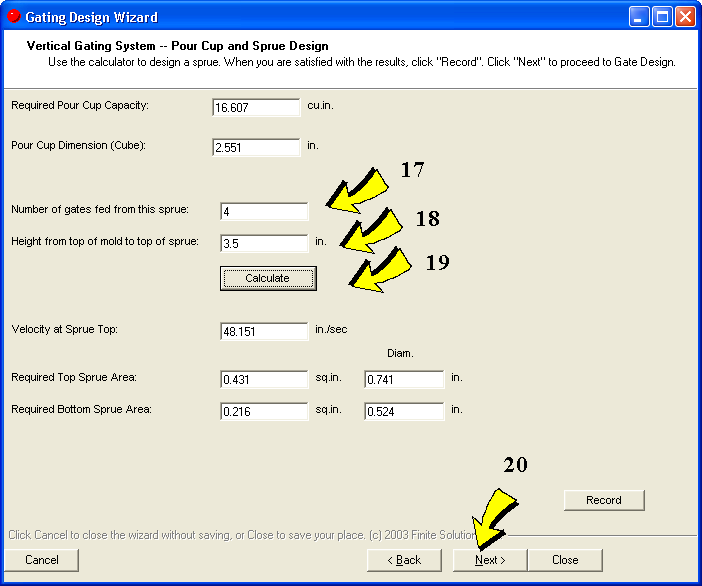The pour cup is designed based on a rule stating that it should have enough volume to accommodate one second of flow, with a minimum dimension of 2.5 inches (63.5 mm). These fields are display only, no data entry is required.

For downsprue design, the program needs to know how many gates are fed from this sprue (thus establishing the flow rate through the sprue) and the height from the top of the mold to the top of the sprue (this latter establishes the velocity, and thus the area, at the sprue top). Be sure to include an allowance for a generous radius at the transition from the bottom of the pour cup to the top of the sprue. Recommended design practice is for the area at the bottom of the sprue to be one-half that at the top.

These are data are entered as Step 17 and Step 18 above. To continue, press the
Calculate button (Step 19). This will display the Required Area and the dimensions at the top and at the bottom of the sprue.

If you wish to record this data for later display in a spreadsheet, press the Record button.

Now, pressing the Next button (Step 20) displays the Runner Design window. This window may be used if you have any horizontal runners in the gating system.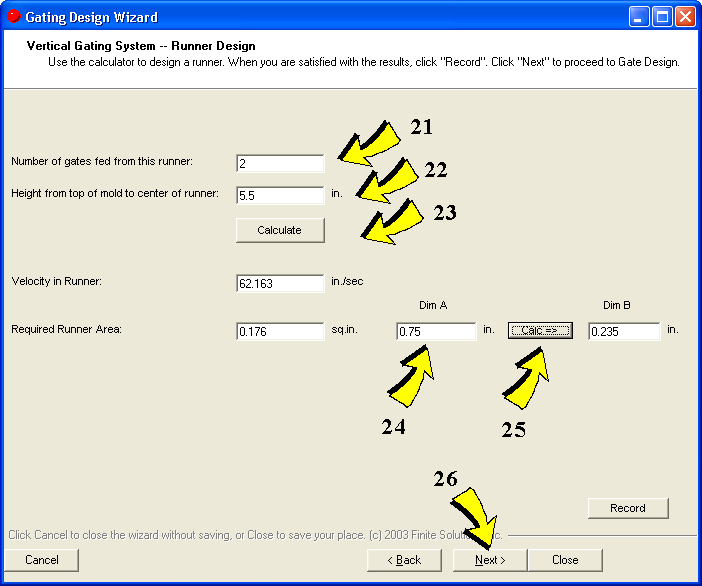For vertical runner design, the program needs to know how many gates are fed from this runner (thus establishing the flow rate through the runner) and the height from the top of the mold to the center of the runner. This latter establishes the velocity (and thus the area) of the runner. These are entered as Step 21 and Step 22 above. Pressing the Calculate button (Step 23) will cause the system to calculate the velocity and the required runner area.

Assuming that the runner is rectangular in cross section, you can enter one dimension (Step 24), press the Calc button (Step 25), and have the system calculate the other dimension of the runner.

If you wish to record this data for later display in a spreadsheet, press the Record button.

Now press Next (Step 26) to proceed to the next window. The following Gate Design window will now appear: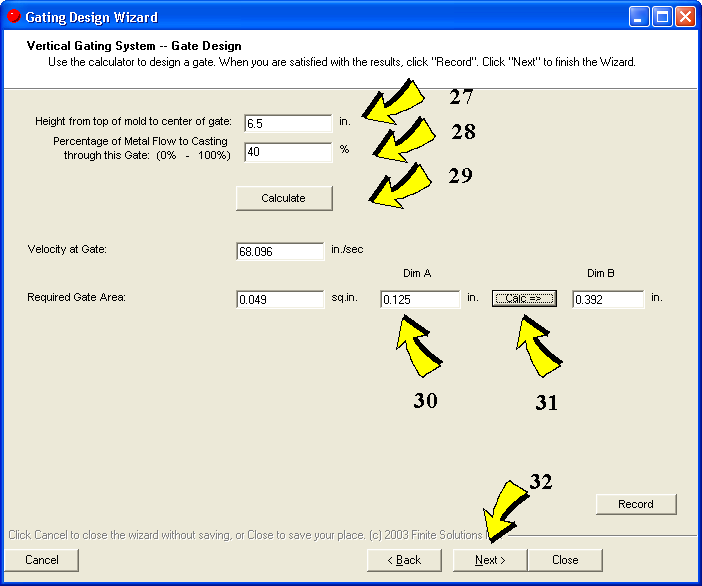For gate design, the program needs to know the height from the top of the mold to the center of this gate (Step 27). If there is more than one gate per casting, then the system will ask what percentage of the flow is to pass through each gate (Step 28). For example, the flow might be divided equally between two gates, which would be 50%-50%, or this could be allocated 40%-60% depending on casting geometry. This data establishes flow rate and velocity at this gate, which makes it possible to calculate required area. This is done by pressing the
Calculate button (Step 29). This will cause the system to calculate the velocity and the required gate area.

Assuming that the gate is rectangular in cross section, you can enter one dimension (Step 30), press the Calc button (Step 31), and have the system calculate the other dimension of the gate.

If you wish to record this data for later display in a spreadsheet, press the Record button.

This procedure can be duplicated for each unique gate within the gating system, so that all gates can be designed using this window.

As an optional final step, you can review all data by pressing the Next button (Step 32) which will bring up the following screen: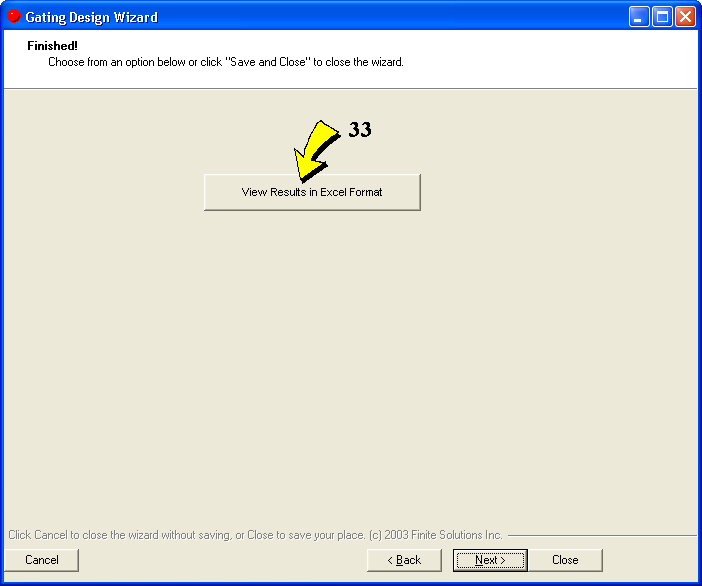By clicking on the button labeled
View Results in Excel Format (Step 33), you can see this data in a spreadsheet format. This assumes that you have Microsoft Excel installed on your computer.